/
Features

# Rotating Objects Using Quaternions

Implementing a floating camera that tracks and rotates about a real-time 3D character is just one of the many reasons you should understand the importance and implementation of quaternions.

Last year may go down in history as The Year of the Hardware Acceleration. Much of the work rasterizing and texture-mapping polygons was off-loaded to dedicated hardware. As a result, we game developers now have a lot of CPU cycles to spare for physics simulation and other features. Some of those extra cycles can be applied to tasks such as smoothing rotations and animations, thanks to quaternions.

Many game programmers have already discovered the wonderful world of quaternions and have started to use them extensively. Several third-person games, including both TOMB RAIDER titles, use quaternion rotations to animate all of their camera movements. Every third-person game has a virtual camera placed at some distance behind or to the side of the player's character. Because this camera goes through different motions (that is, through arcs of a different lengths) than the character, camera motion can appear unnatural and too "jerky" for the player to follow the action. This is one area where quaternions come to rescue.

Another common use for quaternions is in military and commercial flight simulators. Instead of manipulating a plane's orientation using three angles (roll, pitch, and yaw) representing rotations about the x, y, and z axes, respectively, it is much simpler to use a single quaternion.

Many games coming out this year will also feature real-world physics, allowing amazing game play and immersion. If you store orientations as quaternions, it is computationally less expensive to add angular velocities to quaternions than to matrices.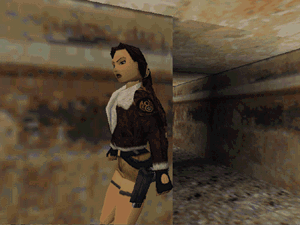Both Tomb Raider titles use quaternion rotations to animate camera movements.

There are many ways to represent the orientation of an object. Most programmers use 3x3 rotation matrices or three Euler angles to store this information. Each of these solutions works fine until you try to smoothly interpolate between two orientations of an object. Imagine an object that is not user controlled, but which simply rotates freely in space (for example, a revolving door). If you chose to store the door's orientations as rotation matrices or Euler angles, you'd find that smoothly interpolating between the rotation matrices' values would be computationally costly and certainly wouldn't appear as smooth to a player's eye as quaternion interpolation.

Trying to correct this problem using matrices or Euler angles, an animator might simply increase the number of predefined (keyed) orientations. However, one can never be sure how many such orientations are enough, since the games run at different frame rates on different computers, thereby affecting the smoothness of the rotation. This is a good time to use quaternions, a method that requires only two or three orientations to represent a simple rotation of an object, such as our revolving door. You can also dynamically adjust the number of interpolated positions to correspond to a particular frame rate.

Before we begin with quaternion theory and applications, let's look at how rotations can be represented. I'll touch upon methods such as rotation matrices, Euler angles, and axis and angle representations and explain their shortcomings and their relationships to quaternions. If you are not familiar with some of these techniques, I recommend picking up a graphics book and studying them.

To date, I haven't seen a single 3D graphics book that doesn't talk about rotations using 4x4 or 3x3 matrices. Therefore, I will assume that most game programmers are very familiar with this technique and I'll just comment on its shortcomings. I also highly recommend that you re-read Chris Hecker's article in the June 1997 issue of the Game Developer ("Physics, Part 4: The Third Dimension," pp.15-26), since it tackles the problem of orienting 3D objects.

Rotations involve only three degrees of freedom (DOF), around the x, y, and z coordinate axes. However, nine DOF (assuming 3x3 matrices) are required to constrain the rotation - clearly more than we need. Additionally, matrices are prone to "drifting," a situation that arises when one of the six constraints is violated and the matrix introduces rotations around an arbitrary axis. Combatting this problem requires keeping a matrix orthonormalized - making sure that it obeys constraints. However, doing so is not computationally cheap. A common way of solving matrix drift relies on the Gram-Schmidt algorithm for conversion of an arbitrary basis into an orthogonal basis. Using the Gram-Schmidt algorithm or calculating a correction matrix to solve matrix drifting can take a lot of CPU cycles, and it has to be done very often, even when using floating point math.

Another shortcoming of rotation matrices is that they are extremely hard to use for interpolating rotations between two orientations. The resulting interpolations are also visually very jerky, which simply is not acceptable in games any more.

You can also use angles to represent rotations around three coordinate axes. You can write this as (q, c, f); simply stated, "Transform a point by rotating it counterclockwise about the z axis by q degrees, followed by a rotation about the y axis by c degrees, followed by a rotation about the x axis by f degrees." There are 12 different conventions that you can use to represent rotations using Euler angles, since you can use any combination of axes to represent rotations (XYZ, XYX, XYY…). We will assume the first convention (XYZ) for all of the presented examples. I will assume that all of the positive rotations are counterclockwise (Figure 1).

Euler angle representation is very efficient because it uses only three variables to represent three DOF. Euler angles also don't have to obey any constraints, so they're not prone to drifting and don't have to be readjusted.

However, there is no easy way to represent a single rotation with Euler angles that corresponds to a series of concatenated rotations. Furthermore, the smooth interpolation between two orientations involves numerical integration, which can be computationally expensive. Euler angles also introduce the problem of "Gimbal lock" or a loss of one degree of rotational freedom. Gimbal lock happens when a series of rotations at 90 degrees is performed; suddenly, the rotation doesn't occur due to the alignment of the axes.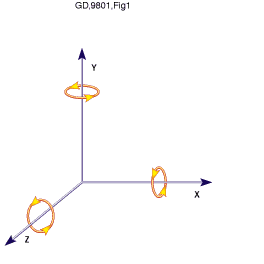Figure 1: Euler angle representation.

For example, imagine that a series of rotations to be performed by a flight simulator. You specify the first rotation to be Q1 around the x axis, the second rotation to be 90 degrees around the y axis, and Q3 to be the rotation around the z axis. If you perform specified rotations in succession, you will discover that Q3 rotation around the z axis has the same effect as the rotation around the initial x axis. The y axis rotation has caused the x and z axes to get aligned, and you have just lost a DOF because rotation around one axis is equivalent to opposite rotation around the other axis. I highly recommend Advanced Animation and Rendering Techniques: Theory and Practice by Alan and Mark Watt (Addison Wesley, 1992) for a detailed discussion of the Gimbal lock problem.

Using an axis and angle representation is another way of representing rotations. You specify an arbitrary axis and an angle (positive if in a counterclockwise direction), as illustrated in Figure 2.

Even though this is an efficient way of representing a rotation, it suffers from the same problems that I described for Euler angle representation (with the exception of the Gimbal lock problem).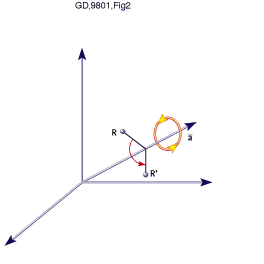In the eighteenth century, W. R. Hamilton devised quaternions as a four-dimensional extension to complex numbers. Soon after this, it was proven that quaternions could also represent rotations and orientations in three dimensions. There are several notations that we can use to represent quaternions. The two most popular notations are complex number notation (Eq. 1) and 4D vector notation (Eq. 2).

w + xi + yj + zk (where i2 = j2 = k2 = -1 and ij = k = -ji with real w, x, y, z)
(Eq. 1)

[w, v] (where v = (x, y, z) is called a "vector" and w is called a "scalar")
(Eq. 2)

I will use the second notation throughout this article. Now that you know how quaternions are represented, let's start with some basic operations that use them.

If q and q´ are two orientations represented as quaternions, you can define the operations in Table 1 on these quaternions.

All other operations can be easily derived from these basic ones, and they are fully documented in the accompanying library, which you can find here. I will also only deal with unit quaternions. Each quaternion can be plotted in 4D space (since each quaternion is comprised of four parts), and this space is called quaternion space. Unit quaternions have the property that their magnitude is one and they form a subspace, S3, of the quaternion space. This subspace can be represented as a 4D sphere. (those that have a one-unit normal), since this reduces the number of necessary operations that you have to perform.

It is extremely important to note that only unit quaternions represent rotations, and you can assume that when I talk about quaternions, I'm talking about unit quaternions unless otherwise specified.

Since you've just seen how other methods represent rotations, let's see how we can specify rotations using quaternions. It can be proven (and the proof isn't that hard) that the rotation of a vector v by a unit quaternion q can be represented as

v´ = q v q-1 (where v = [0, v])
(Eq. 3)

The result, a rotated vector v´, will always have a 0 scalar value for w (recall Eq. 2 earlier), so you can omit it from your computations.

 Table 1. Basic operations using quaternions. Addition: q + q´ = [w + w´, v + v´] Multiplication: qq´ = [ww´ - v · v´, v x v´ + wv´ +w´v] (· is vector dot product and x is vector cross product); Note: qq´ ? q´q Conjugate: q* = [w, -v] Norm: N(q) = w2 + x2 + y2 + z2 Inverse: q-1 = q* / N(q) Unit Quaternion: q is a unit quaternion if N(q)= 1 and then q-1 = q* Identity: [1, (0, 0, 0)] (when involving multiplication) and [0, (0, 0, 0)] (when involving addition)

Today's most widely supported APIs, Direct3D immediate mode (retained mode does have a limited set of quaternion rotations) and OpenGL, do not support quaternions directly. As a result, you have to convert quaternion orientations in order to pass this information to your favorite API. Both OpenGL and Direct3D give you ways to specify rotations as matrices, so a quaternion-to-matrix conversion routine is useful. Also, if you want to import scene information from a graphics package that doesn't store its rotations as a series of quaternions (such as NewTek's LightWave), you need a way to convert to and from quaternion space.

ANGLE AND AXIS. Converting from angle and axis notation to quaternion notation involves two trigonometric operations, as well as several multiplies and divisions. It can be represented as

q = [cos(Q/2), sin(Q /2)v] (where Q is an angle and v is an axis)
(Eq. 4)

EULER ANGLES. Converting Euler angles into quaternions is a similar process - you just have to be careful that you perform the operations in the correct order. For example, let's say that a plane in a flight simulator first performs a yaw, then a pitch, and finally a roll. You can represent this combined quaternion rotation as

q = qyaw qpitch qroll where:
qroll = [cos (y/2), (sin(y/2), 0, 0)]
qpitch = [cos (q/2), (0, sin(q/2), 0)]
qyaw = [cos(f /2), (0, 0, sin(f /2)]
(Eq. 5)

The order in which you perform the multiplications is important. Quaternion multiplication is not commutative (due to the vector cross product that's involved). In other words, changing the order in which you rotate an object around various axes can produce different resulting orientations, and therefore, the order is important.

ROTATION MATRIX. Converting from a rotation matrix to a quaternion representation is a bit more involved, and its implementation can be seen in Listing 1.

Conversion between a unit quaternion and a rotation matrix can be specified as

| 1 - 2y2 - 2z2 2yz + 2wx 2xz - 2wy |
Rm = | 2xy - 2wz 1 - 2x2 - 2z2 2yz - 2wx |
| 2xz + 2wy 2yz - 2wx 1 - 2x2 - 2y2|

(Eq. 6)

It's very difficult to specify a rotation directly using quaternions. It's best to store your character's or object's orientation as a Euler angle and convert it to quaternions before you start interpolating. It's much easier to increment rotation around an angle, after getting the user's input, using Euler angles (that is, roll = roll + 1), than to directly recalculate a quaternion.

Since converting between quaternions and rotation matrices and Euler angles is performed often, it's important to optimize the conversion process. Very fast conversion (involving only nine muls) between a unit quaternion and a matrix is presented in Listing 2. Please note that the code assumes that a matrix is in a right-hand coordinate system and that matrix rotation is represented in a column major format (for example, OpenGL compatible).

Listing 1: Matrix to quaternion code.

```MatToQuat(float m, QUAT * quat)
{
float  tr, s, q;
int    i, j, k;
int nxt = {1, 2, 0};
tr = m + m + m;
// check the diagonal
if (tr > 0.0) {
s = sqrt (tr + 1.0);
quat->w = s / 2.0;
s = 0.5 / s;
quat->x = (m - m) * s;
quat->y = (m - m) * s;
quat->z = (m - m) * s;
} else {
// diagonal is negative
i = 0;
if (m > m) i = 1;
if (m > m[i][i]) i = 2;
j = nxt[i];
k = nxt[j];
s = sqrt ((m[i][i] - (m[j][j] + m[k][k])) + 1.0);
q[i] = s * 0.5;
if (s != 0.0) s = 0.5 / s;
q = (m[j][k] - m[k][j]) * s;
q[j] = (m[i][j] + m[j][i]) * s;
q[k] = (m[i][k] + m[k][i]) * s;
quat->x = q;
quat->y = q;
quat->z = q;
quat->w = q;
}
}
```

If you aren't dealing with unit quaternions, additional multiplications and a division are required. Euler angle to quaternion conversion can be coded as shown in Listing 3.

One of the most useful aspects of quaternions that we game programmers are concerned with is the fact that it's easy to interpolate between two quaternion orientations and achieve smooth animation. To demonstrate why this is so, let's look at an example using spherical rotations. Spherical quaternion interpolations follow the shortest path (arc) on a four-dimensional, unit quaternion sphere. Since 4D spheres are difficult to imagine, I'll use a 3D sphere (Figure 3) to help you visualize quaternion rotations and interpolations.

Let's assume that the initial orientation of a vector emanating from the center of the sphere can be represented by q1 and the final orientation of the vector is q3. The arc between q1 and q3 is the path that the interpolation would follow. Figure 3 also shows that if we have an intermediate position q2, the interpolation from q1 -> q2 -> q3 will not necessarily follow the same path as the q1 ->q3 interpolation. The initial and final orientations are the same, but the arcs are not.

Quaternions simplify the calculations required when compositing rotations. For example, if you have two or more orientations represented as matrices, it is easy to combine them by multiplying two intermediate rotations.

R = R2R1 (rotation R1 followed by a rotation R2)
(Eq. 7)

Listing 2: Quaternion-to-matrix conversion. ([07.30.02] Editor's Note: the following QuatToMatrix function originally was published with a bug -- it reversed the row/column ordering. This is the correct version. Thanks to John Ratcliff and Eric Haines for pointing this out.)

QuatToMatrix(QUAT * quat, float m){

float wx, wy, wz, xx, yy, yz, xy, xz, zz, x2, y2, z2;

// calculate coefficients
x2 = quat->x + quat->x; y2 = quat->y + quat->y;
z2 = quat->z + quat->z;
xx = quat->x * x2; xy = quat->x * y2; xz = quat->x * z2;
yy = quat->y * y2; yz = quat->y * z2; zz = quat->z * z2;
wx = quat->w * x2; wy = quat->w * y2; wz = quat->w * z2;

m = 1.0 - (yy + zz); m = xy - wz;
m = xz + wy; m = 0.0;

m = xy + wz; m = 1.0 - (xx + zz);
m = yz - wx; m = 0.0;

m = xz - wy; m = yz + wx;
m = 1.0 - (xx + yy); m = 0.0;

m = 0; m = 0;
m = 0; m = 1;

}

This composition involves 27 multiplications and 18 additions, assuming 3x3 matrices. On the other hand, a quaternion composition can be represented as

q = q2q1 (rotation q1 followed by a rotation q2)
(Eq. 8)

As you can see, the quaternion method is analogous to the matrix composition. However, the quaternion method requires only eight multiplications and four divides (Listing 4), so compositing quaternions is computationally cheap compared to matrix composition. Savings such as this are especially important when working with hierarchical object representations and inverse kinematics.

Now that you have an efficient multiplication routine, see how can you interpolate between two quaternion rotations along the shortest arc. Spherical Linear intERPolation (SLERP) achieves this and can be written as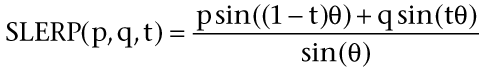(Eq. 9)

where pq = cos(q) and parameter t goes from 0 to 1. The implementation of this equation is presented in Listing 5. If two orientations are too close, you can use linear interpolation to avoid any divisions by zero.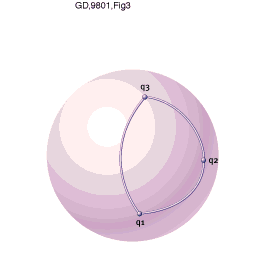Figure 3. Quaternion rotations.

Listing 3: Euler-to-quaternion conversion.

```EulerToQuat(float roll, float pitch, float yaw, QUAT * quat)
{
float cr, cp, cy, sr, sp, sy, cpcy, spsy;
// calculate trig identities
cr = cos(roll/2);
cp = cos(pitch/2);
cy = cos(yaw/2);
sr = sin(roll/2);
sp = sin(pitch/2);
sy = sin(yaw/2);
cpcy = cp * cy;
spsy = sp * sy;
quat->w = cr * cpcy + sr * spsy;
quat->x = sr * cpcy - cr * spsy;
quat->y = cr * sp * cy + sr * cp * sy;
quat->z = cr * cp * sy - sr * sp * cy;
}
```

The basic SLERP rotation algorithm is shown in Listing 6. Note that you have to be careful that your quaternion represents an absolute and not a relative rotation. You can think of a relative rotation as a rotation from the previous (intermediate) orientation and an absolute rotation as the rotation from the initial orientation. This becomes clearer if you think of the q2 quaternion orientation in Figure 3 as a relative rotation, since it moved with respect to the q1 orientation. To get an absolute rotation of a given quaternion, you can just multiply the current relative orientation by a previous absolute one. The initial orientation of an object can be represented as a multiplication identity [1, (0, 0, 0)]. This means that the first orientation is always an absolute one, because

q = qidentity q
(Eq. 10)

Listing 4: Efficient quaternion multiplication.

```QuatMul(QUAT *q1, QUAT *q2, QUAT *res){
float A, B, C, D, E, F, G, H;
A = (q1->w + q1->x)*(q2->w + q2->x);
B = (q1->z - q1->y)*(q2->y - q2->z);
C = (q1->w - q1->x)*(q2->y + q2->z);
D = (q1->y + q1->z)*(q2->w - q2->x);
E = (q1->x + q1->z)*(q2->x + q2->y);
F = (q1->x - q1->z)*(q2->x - q2->y);
G = (q1->w + q1->y)*(q2->w - q2->z);
H = (q1->w - q1->y)*(q2->w + q2->z);
res->w = B + (-E - F + G + H) /2;
res->x = A - (E + F + G + H)/2;
res->y = C + (E - F + G - H)/2;
res->z = D + (E - F - G + H)/2;
}
```

As I stated earlier, a practical use for quaternions involves camera rotations in third-person-perspective games. Ever since I saw the camera implementation in TOMB RAIDER, I've wanted to implement something similar. So let's implement a third-person camera (Figure 4).

To start off, let's create a camera that is always positioned above the head of our character and that points at a spot that is always slightly above the character's head. The camera is also positioned d units behind our main character. We can also implement it so that we can vary the roll (angle q in Figure 4) by rotating around the x axis.

As soon as a player changes the orientation of the character, you rotate the character instantly and use SLERP to reorient the camera behind the character (Figure 5). This has the dual benefit of providing smooth camera rotations and making players feel as though the game responded instantly to their input.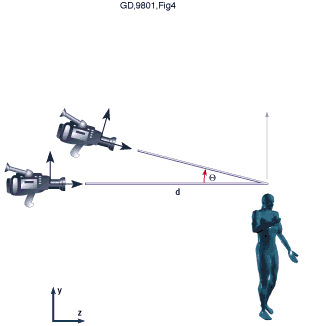Figure 4. Third-person camera.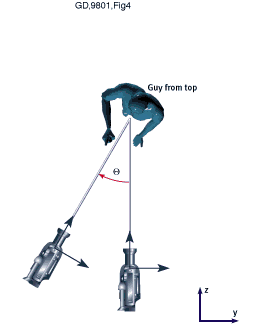Figure 5. Camera from top.

You can set the camera's center of rotation (pivot point) as the center of the object it is tracking. This allows you to piggyback on the calculations that the game already makes when the character moves within the game world.

Note that I do not recommend using quaternion interpolation for first-person action games since these games typically require instant response to player actions, and SLERP does take time.

However, we can use it for some special scenes. For instance, assume that you're writing a tank simulation. Every tank has a scope or similar targeting mechanism, and you'd like to simulate it as realistically as possible. The scoping mechanism and the tank's barrel are controlled by a series of motors that players control. Depending on the zoom power of the scope and the distance to a target object, even a small movement of a motor could cause a large change in the viewing angle, resulting in a series of huge, seemingly disconnected jumps between individual frames. To eliminate this unwanted effect, you could interpolate the orientation according to the zoom and distance of object. This type of interpolation between two positions over several frames helps dampen the rapid movement and keeps players from becoming disoriented.

Another useful application of quaternions is for prerecorded (but not prerendered) animations. Instead of recording camera movements by playing the game (as many games do today), you could prerecord camera movements and rotations using a commercial package such as Softimage 3D or 3D Studio MAX. Then, using an SDK, export all of the keyframed camera/object quaternion rotations. This would save both space and rendering time. Then you could just play the keyframed camera motions whenever the script calls for cinematic scenes.

Listing 5: SLERP implementation.

```QuatSlerp(QUAT * from, QUAT * to, float t, QUAT * res)
{
float           to1;
double        omega, cosom, sinom, scale0, scale1;
// calc cosine
cosom = from->x * to->x + from->y * to->y + from->z * to->z
+ from->w * to->w;
if ( cosom <0.0 ){ cosom = -cosom; to1 = - to->x;
to1 = - to->y;
to1 = - to->z;
to1 = - to->w;
} else  {
to1 = to->x;
to1 = to->y;
to1 = to->z;
to1 = to->w;
}
// calculate coefficients
if ( (1.0 - cosom) > DELTA ) {
// standard case (slerp)
omega = acos(cosom);
sinom = sin(omega);
scale0 = sin((1.0 - t) * omega) / sinom;
scale1 = sin(t * omega) / sinom;
} else {
// "from" and "to" quaternions are very close
//  ... so we can do a linear interpolation
scale0 = 1.0 - t;
scale1 = t;
}
// calculate final values
res->x = scale0 * from->x + scale1 * to1;
res->y = scale0 * from->y + scale1 * to1;
res->z = scale0 * from->z + scale1 * to1;
res->w = scale0 * from->w + scale1 * to1;
}
```

After reading Chris Hecker's columns on physics last year, I wanted to add angular velocity to a game engine on which I was working. Chris dealt mainly with matrix math, and because I wanted to eliminate quaternion-to-matrix and matrix-to-quaternion conversions (since our game engine is based on quaternion math), I did some research and found out that it is easy to add angular velocity (represented as a vector) to a quaternion orientation. The solution (Eq. 11) can be represented as a differential equation.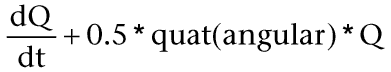(Eq. 11)

where quat(angular) is a quaternion with a zero scalar part (that is, w = 0) and a vector part equal to the angular velocity vector. Q is our original quaternion orientation.

To integrate the above equation (Q + dQ/dt), I recommend using the Runge-Kutta
order four method. If you are using matrices, the Runge-Kutta order five method achieves better results within a game. (The Runge-Kutta method is a way of integrating differential equations. A complete description of the method can be found in any elementary numerical algorithm book, such as Numerical Recipes in C. It has a complete section devoted to numerical, differential integration.) For a complete derivation of angular velocity
integration, consult Dave Baraff's SIGGRAPH tutorials.

Quaternions can be a very efficient and extremely useful method of storing and performing rotations, and they offer many advantages over other methods. Unfortunately, they are also impossible to visualize and completely unintuitive. However, if you use quaternions to represent rotations internally, and use some other method (for example, angle-axis or Euler angles) as an immediate representation, you won't have to visualize them.

Nick Bobick is a game developer at Caged Entertainment Inc. and he is currently working on a cool 3D game. He can be contacted at [email protected]. The author would like to thank Ken Shoemake for his research and publications. Without him, this article would not have been possible.

### Latest Jobs

#### Disbelief

Hybrid, Cambridge, MA or Chicago, IL
5.31.23

Remote (United States)
5.18.23
Senior Gameplay Engineer

#### High Fidelity, Inc.

Remote
6.2.23
Game Interaction Designer

#### Fred Rogers Productions

Hybrid (424 South 27th Street, Pittsburgh, PA, USA
5.19.23
Producer - Games & Websites
More Jobs

#### Game Developer Job Board

Browse open positions across the game industry or recruit new talent for your studio